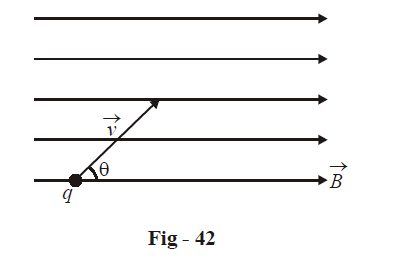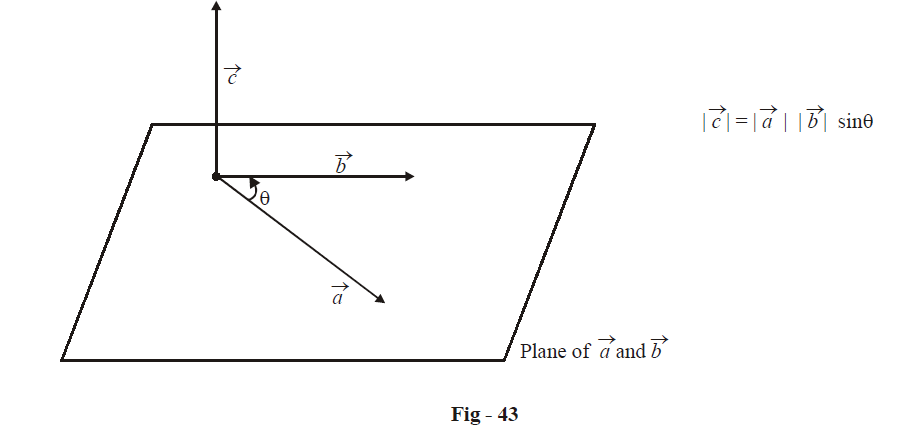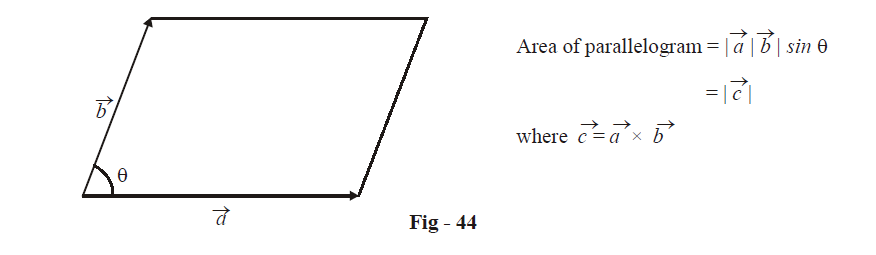# Vector Cross Product

Go back to  'Vectors and 3-D Geometry'

The dot product discussed in the previous section, was introduced through the requirement that arose in calculating the work done by a given force  $$\vec F$$ when the point of application of the force is displaced by a certain amount given by $$\vec s$$ :

$W = \vec F \cdot \vec s$

In this section, we’ll see that another form of vector product exists and is extremely useful to discuss many different physical phenomena; this product is called the cross product. The cross product of $$\vec a\,\,{\text{and}}\,\,\vec b$$  is another vector $$\vec c$$  and the relation is represented as

$\vec c = \vec a \times \vec b$

Let us, through a physical example, understand what the cross product means.

Consider a horizontal magnetic field, which we can represent by $$\vec B$$  , and a charge q projected into this field with a velocity $$\vec v$$(at an angle  $$\theta$$ with the horizontal).Experiments show that the force $$\vec F$$ acting on this particle

(a) is perpendicular to the plane of $$\vec v$$ and $$\vec B$$  and goes into the plane for the figure above.

(b) increases with increase in $$\left| {\vec v} \right|\;\;{\text{and}}\;\;\left| {\vec B} \right|$$.

(c) is such that its magnitude increases as $$\theta$$ goes from $$0\;\;{\text{to}}\;\frac{\pi }{2}$$. In fact, when $$\vec v$$ and $$\vec B$$ are parallel, the force on the particle is zero. For fixed magnitudes of $$\vec v$$ and $$\vec B$$, the force is the maximum when \begin{align}\theta = \frac{\pi }{2}\end{align} .

(d) increases with increase in charge.

This suggests the dependence

$\left| {\vec F} \right|\; \propto q\;\left| {\vec v} \right|\;\left| {\vec B} \right|\;\sin \theta$

which has been confirmed experimentally. In fact, the relation is (exactly),

$\left| {\vec F} \right| = q\;\left| {\vec v} \right|\;\left| {\vec B} \right|\;\sin \theta$

The direction of $$\vec F$$ is found out to satisfy the right hand thumb rule. Holding out your thumb use your right hand fingers to map out the rotation from $$\vec v$$ to $$\vec B$$. The direction of  $$\vec F$$ is given by the direction in which the thumb points.

Now, since $$\vec F$$  is a vector with direction perpendicular to both $$\vec v$$ and $$\vec B$$, we write the expression for $$\vec F$$ as

$\boxed{\vec F = q(\vec v \times \vec B)}$

where the vector  $$\vec v \times \vec B$$, the cross product of $$\vec v$$ and $$\vec B$$ , is understood to be a vector such that its magnitude is $$\left| {\vec v} \right|\left| {\vec B} \right|\sin \theta .$$ and its direction is given by the right hand thumb rule

In general, the cross product of $$\vec a\,\,and\,\,\vec b$$ , i.e.$$\vec c = \vec a \times \vec b$$ is a vector with magnitude $$\left| {\vec a} \right|\;\left| {\vec b} \right|\sin {{\theta }}$$( $${{\theta }}$$ being the angle between $$\vec a\,\,and\,\,\vec b$$ ) and direction perpendicular to the plane of $$\vec a\,\,and\,\,\vec b$$ such that $$\vec a\,\,,\,\,\vec b$$ and this direction form a right handed system.It is important to keep in mind that the cross product is a vector; the dot product was a scalar. The cross product is also referred to as the vector product.

The cross product of  $$\vec a\,\,{\text{and}}\,\,\vec b$$ , say $$\vec c$$, has an interesting geometrical interpretation. Since $$\left| {\vec c} \right| = \left| {\vec a} \right|\;\left| {\vec b} \right|\;\sin \theta ,\,\,\left| {\vec c} \right|$$ represents the area of the parallelogram with adjacent sides $$\vec a\,\,{\text{and}}\,\,\vec b$$ :In fact, the area of the parallelogram can itself be treated as a vector (as it is in physical phenomena):

$\vec A = \vec a \times \vec b$

The area of the triangle formed with $$\vec a\,\,{\text{and}}\,\,\vec b$$ as two sides is simply \begin{align}\frac{1}{2}\left| {\vec A} \right| = \frac{1}{2}\;\left| {\vec a \times \vec b} \right|\end{align} .

Vectors
grade 11 | Questions Set 1
Vectors